# Determine the optimal combination of overlapping discounts

When discounts overlap, you must determine the combination of overlapping discounts that will produce the lowest transaction total or the highest total discount. When the discount amount varies according to the price of the products that are purchased, such as in the common "Buy 1, get 1 X percent off" (BOGO) retail discount, this process becomes an issue of combinatorial optimization.

This article applies to Microsoft Dynamics AX 2012 R3 with KB 3105973 (released November 2, 2015) or later, and to Dynamics 365 Commerce. To determine the combination overlapping discounts to apply in a timely manner, we have introduced a method for applying overlapping discounts. We call this new method marginal value ranking. Marginal value ranking is used when the time that is required in order to evaluate the possible combinations of overlapping discounts exceeds a threshold that is configurable on the Commerce parameters page. In the marginal value ranking method, a value is calculated for each overlapping discount by using the discount's value on the shared products. The overlapped discounts are then applied from the highest relative value to the lowest relative value. For details about the new method, see the "Marginal value" section, later in this article. Marginal value ranking isn't used when the discount amounts for a product aren't affected by another product in the transaction. For example, this method isn't used for two simple discounts, or for a simple discount and a single product quantity discount.

## Discount examples

You can create an unlimited number of discounts on a common set of products. However, because there is no limit, performance issues can occur when you try to calculate the discounts that should be used on the various products. The following examples illustrate this issue in more detail. In example 1, we start with two products and two overlapping discounts. Then, in example 2, we show how the issue evolves as more products are added.

### Example 1: Two products and two discounts

In this example, two products are required in order to qualify for each discount, and the discounts can't be combined. The discounts in this example are Best price discounts. Both products qualify for both discounts. Here are the two discounts.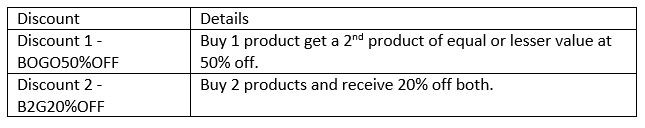For any two products, the better of these two discounts depends on the prices of the two products. When the price of both products is equal or almost equal, discount 1 is better. When the price of one product is significantly less than the price of the other product, discount 2 is better. Here is the mathematical rule for evaluating these two discounts against each other.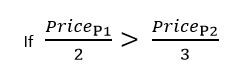Note

When the price of product 1 is equal to two-thirds of the price of product 2, the two discounts are equal. In this example, the effective discount percentage for discount 1 varies from a few percent (when the prices of the two products are far apart) to a maximum of 25 percent (when the two products have the same price). The effective discount percentage for discount 2 is fixed. It's always 20 percent. Because the effective discount percentage for discount 1 has a range that can be more than or less than discount 2, the best discount depends on the prices of the two products that must be discounted. In this example, the calculation is completed quickly, because only two discounts are applied on only two products. There are only two possible combinations: one application of discount 1 or one application of discount 2. There are no permutations to calculate. The value of each discount is calculated by using both products, and the best discount is used.

### Example 2: Four products and two discounts

Next, we will use four products and the same two discounts. All four products qualify for both discounts. There are twelve possible combinations. In the end, two discounts will be applied to the transaction in one of three combinations: two applications of discount 1, two applications of discount 2, or one application of discount 1 and one application of discount 2. To illustrate the possible combinations, we will look at two different sets of four products that have different prices:

• All four products have the same price, \$15.00. In this case, the best discount combination is two applications of discount 1. Two products will be full price, and two will be 50 percent off. The discounted total for the transaction is \$45 (15 + 15 + 7.50 + 7.50), which is \$15 (25 percent) off the undiscounted total of \$60. Discount 2 is only \$12 (20 percent).
• Two products are \$20 each, one product is \$15, and one product is \$5. In this case, the best discount combination is one application of discount 2 and one application of discount 1. The following tables illustrates the discounts.

To read the tables, use one product from a row and one product from a column. For example, in the table for discount 1, when you combine the two \$20 products, you get \$10 off. In the table for discount 2, when you combine the \$15 product and the \$5 product, you get \$4 off.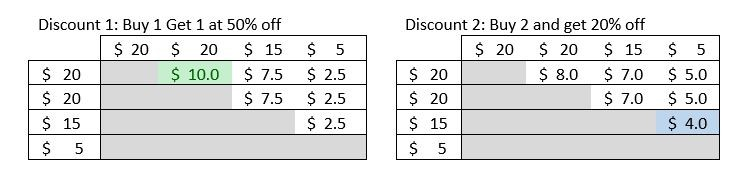First, we find the largest discount that is available from any two products by using either discount. The two tables show the discount amount for all combinations of the two products. The shaded portions of the tables represent either cases where a product is paired with itself, which we can't do, or a reverse pairing of two products that produces the same discount amount and can be ignored. By looking at the tables, you can see that discount 1 for the two \$20 items is the largest discount that is available for either discount on all four products. (This discount is highlighted in green in the first table.) That leaves only the \$15 product and the \$5 product. By looking at the two tables again, you can see that, for these two products, discount 1 gives a \$2.50 discount, whereas discount 2 gives a \$4 discount. Therefore, we select discount 2. The total discount is \$14. To make this discussion easier to visualize, here are two additional tables that show the effective discount percentage for all possible two-product combinations for both discount 1 and discount 2. Only half the list of combinations is included, because, for these two discounts, the order in which the two products are put into the discount doesn't matter. The highest effective discount (25 percent) is highlighted in green, and the lowest effective discount (10 percent) is highlighted in red.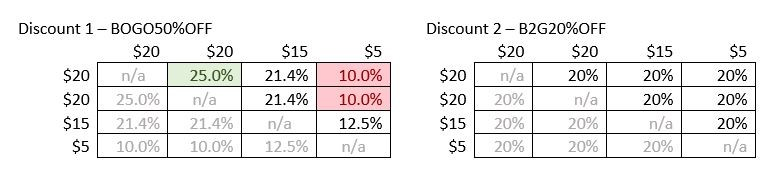Note

When prices vary, and two or more discount compete, the only way to guarantee the best combination of discounts is to evaluate both discounts and compare them.

## Total possible combinations

This section continues the example from the previous section. We will add more products and another discount, and see how many combinations must be calculated and compared. The following table shows the number of possible discount combinations as the product quantity increases. The table shows what happens both when there are two overlapping discounts, as in the previous example, and when there are three overlapping discounts. The number of possible discount combinations that must be evaluated soon exceeds what even a fast computer can calculate and compare quickly enough to be acceptable for retail transactions.When even larger quantities or more overlapping discounts are applied, the total number of possible discount combinations quickly goes into the millions, and the time that is required in order to evaluate and select the best possible combination quickly becomes noticeable. Some optimizations have been done in the price engine to reduce the total number of combinations that must be evaluated. However, because the number overlapping discounts and the quantities in a transaction aren't limited, a large number of combinations will always have to be evaluated whenever there are overlapping discounts. This issue is the issue that the marginal value ranking method addresses.

## Marginal value method

To resolve the issue of an exponentially increasing number of combinations that must be evaluated, an optimization exists that calculates the value per shared product of each discount on the set of products that two or more discounts can be applied to. We refer to this value as the marginal value of the discount for the shared products. The marginal value is the average per product increase in the total discount amount when the shared products are included in each discount. The marginal value is calculated by taking the total discount amount (DTotal), subtracting the discount amount without the shared products (DMinus\ Shared), and dividing that difference by the number of shared products (ProductsShared).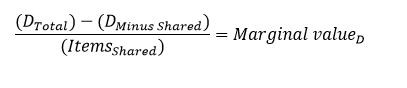After the marginal value of each discount on a shared set of products is calculated, the discounts are applied to the shared products in order, exhaustively, from highest marginal value to lowest marginal value. For this method, all remaining discount possibilities aren't compared every time after a single instance of a discount is applied. Instead, the overlapping discounts are compared one time and then applied in order. No additional comparisons are done. You can configure the threshold to switch to the marginal value method on the Discount tab of the Commerce parameters page. The acceptable time to calculate the total discount varies across retail industries. However, this time generally falls in the range of tens of milliseconds to one second.

## Help us understand

We want to learn more about how people use Microsoft's custom Help toolkit. Take the survey (in English) and help us understand: https://forms.office.com/r/A4cUJgjkD1.